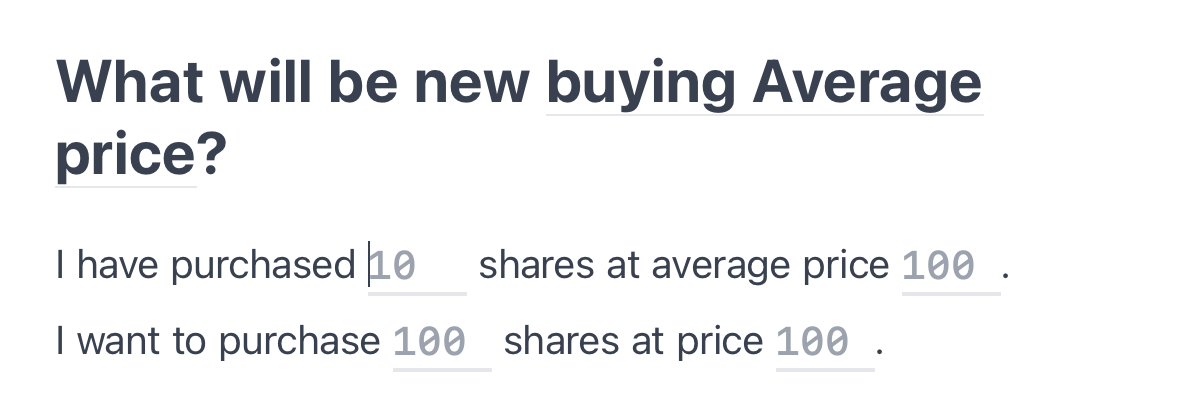# What is the Average Buying Price of a stock?

## by Scripo

Average Buying Price of a stock is "the total amount invested in the stock" divided by "the total number of shares purchased of the stock".

## Average Buying Price using Arithmetic mean

One way of calculating Average Buying Price is using the arithmetic mean. In a sense, Average Buying Price is the arithmetic mean of individual share's price that you have bought over time.

### Example 1

Say you have bought three shares of a company at prices: `\$1`, `\$2`, and `\$3`, then Average Buying Price would be:

You can say that you paid `\$2` for each of the three shares.

### Example 2

Say you have bought five shares: three shares for `\$2` and the rest for `\$4`, then Average Buying Price would be:

You have purchased five shares for `\$2.8` each.

## Average Buying Price using Weighted Average

In practice, it is not easy to add up individual share prices. It can be simplified using a mathematical formulation called Weighted Average, which takes purchased quantity and the price (for which many shares are collected).

- p1 is the price at share unit - q1 is the number of shares bought at price p1 so on

In a grand sense, you reach back to the invested amount of a transaction by multiplying purchased units with unit price. Weighted Average helps you calculate the average price over multiple transactions.

### In practice

Let's understand Weighted Average formulation with an example:
Say you have bought five shares: three shares for `\$2` and the rest for `\$4`, then Buying Average Price would be:

## Significance of Average Buying Price

Average Buying Price acts as a reference for calculating your profit & loss. Selling or purchasing some shares requires calculating the new Average Price. Understanding how the new price is calculated helps in making well-informed decisions.

Let's understand it with an example:

Extending example 2, let's say you buy five additional shares for `\$3`; you need to recalculate the average price:

You don't need to add the individual share price to your calculation; instead, you can use the previously calculated average price, i.e., `\$2.8`

You don't need to remember or apply the formula on your own; [Scripo](https://scripo.co) has created a tool for you for such calculation.Tap to use tool

## Summary

In simple understanding, Average Buying price is the total amount invested divided by the total number of shares purchased. It acts as a reference price for communication and calculating profit & loss.

In real life, you don't buy shares in a single transaction; you might need to estimate the "future buying average" before you invest more in the stock. So, understanding how average buying calculation works can save you from blunders.